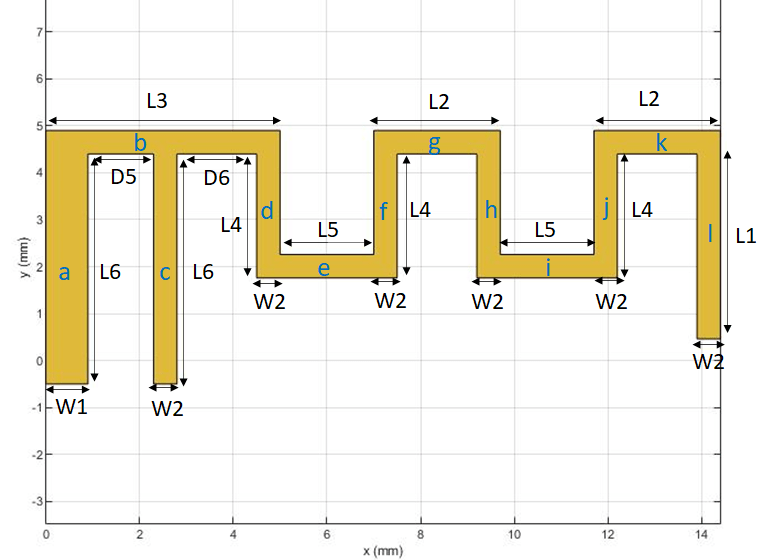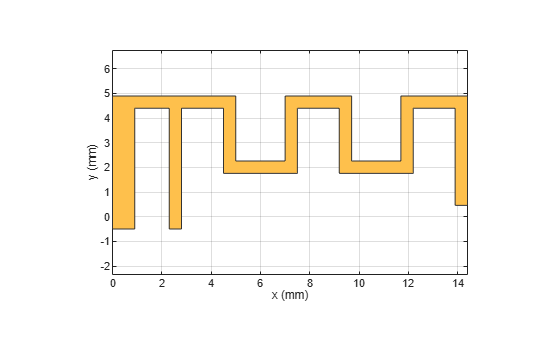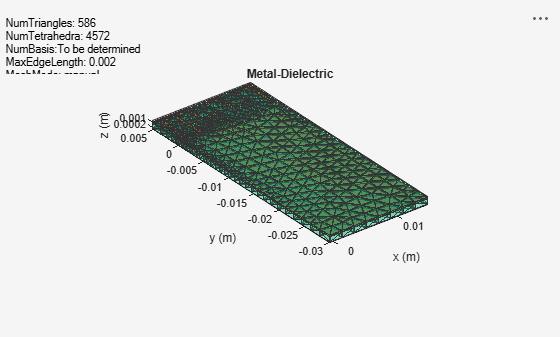# Design PCB Antenna for USB Dongle and BLE Applications at 2.4 GHz

This example shows how to design and analyze a small PCB antenna usually used on a USB dongle and in BLE applications. The antenna design and dimensions are as specified in the application note in . This antenna design requires no more than 15.2 by 5.7 mm of space and ensures a Voltage Standing Wave Ratio (VSWR) of less than 2 across the 2.4 GHz Industrial, Scientific, and Medical frequency(ISM) band when you connect antenna to a 50 ohm source.### Create Variables

Assign the variables to create the top metal layer.

```L1 = 3.94e-3; L2 = 2.70e-3; L3 = 5e-3; L4 = 2.64e-3; L5 = 2e-3; L6 = 4.90e-3; W1 = 0.90e-3; W2 = 0.5e-3; D4 = 0.5e-3; D5 = 1.4e-3; D6 = 1.70e-3; Lgp = 15.2e-3; % Length of the Groundplane Wgp = 30e-3; % Width of the Groundplane LBS = 15.2e-3; % Length for the Board Shape WBS = 5.2e-3; % Width of the Board Shape```

### Create Geometry

Use the `antenna.Rectangle` object to create all the rectangular sections as shown in the schematic above.

```a = antenna.Rectangle('Length',W1,'Width',L6,'Center',[W1/2,L6/2-D4]); b = antenna.Rectangle('Length',L3,'Width',W2,'Center',[L3/2,W2/2+L6-D4]); c = antenna.Rectangle('Length',W2,'Width',L6,'Center',[W2/2+D5+W1,L6/2-D4]); d = antenna.Rectangle('Length',W2,'Width',L4,'Center',[W2/2+W1+D5+W2+D6,-L4/2+L6-D4]); e = antenna.Rectangle('Length',L5,'Width',W2,'Center',[L5/2+L3,-W2/2+L6-L4]); f = antenna.Rectangle('Length',W2,'Width',L4,'Center',[W2/2+W1+D5+W2+D6+W2+L5,-L4/2+L6-D4]); g = antenna.Rectangle('Length',L2,'Width',W2,'Center',[L2/2+W1+D5+W2+D6+W2+L5,W2/2+L6-D4]); h = antenna.Rectangle('Length',W2,'Width',L4,'Center',[W2/2+W1+D5+D6+W2+L5+L2,-L4/2+L6-D4]); i = antenna.Rectangle('Length',L5,'Width',W2,'Center',[L5/2+L3+L5+L2,-W2/2+L6-L4]); j = antenna.Rectangle('Length',W2,'Width',L4,'Center',[W2/2+W1+D5+W2+D6+W2+L5+L2+L5,-L4/2+L6-D4]); k = antenna.Rectangle('Length',L2,'Width',W2,'Center',[L2/2+W1+D5+W2+D6+W2+L5+L2+L5,W2/2+L6-D4]); l = antenna.Rectangle('Length',W2,'Width',L1,'Center',[W1+D5+W2+D6+W2/2+L5+L2+L5+L2,-L1/2+L6-D4]);```

Join all the rectangles and visualize the shape.

```Newobj = a+b+c+d+e+f+g+h+i+j+k+l; figure; show(Newobj)```### Design PCB Board

Design the PCB board as per the specifications in section 4.3 of the application note in . Information given in  is also given below.

1-2 LAYER PCB 0.25 MM NOMINAL

2-3 LAYER PCB 0.50 MM NOMINAL

3-4 LAYER PCB 0.25 MM NOMINAL

THICKNESS FR4 WITH 35um Cu PER LAYER

Dielectric constant for FR4 is 4.5

The ground plane dimensions are taken as 30 mm and the dielectric substrate is FR4 with a total board height of 1 mm. The PCB antenna has four layers and the details of the layers are given below.

2) Dielectric Layer- 0.25 mm

3) Ground Layer - Conductor

4) Dielectric Layer- 0.75 mm - This height is taken after combining the 2-3 layer and 3-4 layer as given in the PCB description

Define the dielectric substrate for the antenna. The antenna has the top radiating layer followed by a dielectric layer of 0.25 mm in thickness. The third layer is the ground layer, which is followed by another dielectric layer 0.75 mm in thickness.

```d = dielectric('FR4'); d.Thickness = 0.25e-3; d.EpsilonR = 4.5; d1 = dielectric('FR4'); d1.Thickness = 0.75e-3; d1.EpsilonR = 4.5;```

Use the `pcbStack` object to create the PCB stack of the antenna. Use the `antenna.Rectangle` object to create the board and the ground plane. Assign the board, dielectrics, and ground plane to the `Layers` property of `pcbStack`. Set the `FeedLocations` and `Vialocations` property to create a feed and via from the first layer to the third layer, and set the `BoardThickness` to 1 mm.

Use the `show` function to visualize the antenna.

```ant = pcbStack; boardshape = antenna.Rectangle('Length',LBS,'Width',WBS+Wgp,'Center',[LBS/2-0.5e-3,(WBS-Wgp)/2]); ant.BoardShape = boardshape; gnd = antenna.Rectangle('Length',Lgp,'Width',Wgp,'Center',[Lgp/2-0.5e-3,-Wgp/2]); ant.Layers = {Newobj,d,gnd,d1}; ant.FeedDiameter = W2/2; ant.ViaDiameter = W1/4; ant.FeedLocations = [W1+1.4e-3+W2/2,-D4/2,3,1]; ant.ViaLocations = [W1/2,-D4/2,3,1]; ant.BoardThickness = 1e-3; ant.FeedViaModel = 'square'; ant.Conductor = metal('Copper'); ant.Conductor.Thickness = 35e-6; figure; show(ant)```### Analyze Antenna Performance

Use the `sparameters` function to calculate the S-parameters and plot them using the `rfplot` function.

```spar = sparameters(ant,linspace(2e9,3e9,21)); figure; rfplot(spar);```Use the `mesh` function with a `MaxEdgeLength` of 1.2 mm to generate a denser mesh, to ensure around 50 triangles per wavelength.

```figure; mesh(ant,'MaxEdgeLength',1.2e-3);```Use the `sparameters` function to calculate the S-parameters for the improved mesh and plot them using the `rfplot` function.

```spar = sparameters(ant,linspace(2.2e9,2.7e9,11)); figure; rfplot(spar)```Use the `pattern` function to plot the 3-D pattern of the antenna.

```figure; pattern(ant,2.4e9)```### References

 Andersen, Audun. “Small Size 2.4 GHz PCB Antenna .” Texas Instruments. Accessed January 14, 2022. https://www.ti.com/lit/an/swra117d/swra117d.pdf.